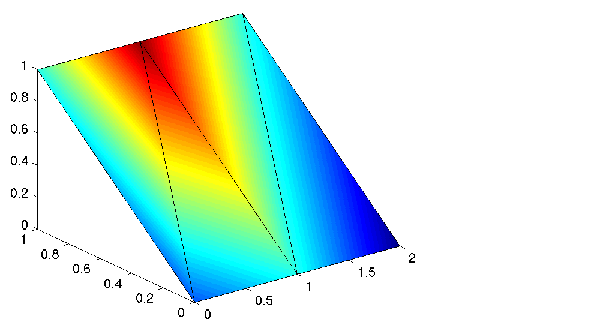MATLAB Function Referencefill3

Filled three-dimensional polygons

Syntax

• fill3(X,Y,Z,C)
fill3(X,Y,Z,ColorSpec)
fill3(X1,Y1,Z1,C1,X2,Y2,Z2,C2,...)
fill3(...,'PropertyName',PropertyValue)
h = fill3(...)

Description

fill3(X,Y,Z,C) fills three-dimensional polygons. X, Y, and Z triplets specify the polygon vertices. If X, Y, or Z is a matrix, fill3 creates n polygons, where n is the number of columns in the matrix. fill3 closes the polygons by connecting the last vertex to the first when necessary.

C specifies color, where C is a vector or matrix of indices into the current colormap. If C is a row vector, length(C) must equal size(X,2) and size(Y,2); if C is a column vector, length(C) must equal size(X,1) and size(Y,1).

fill3(X,Y,Z,ColorSpec) fills three-dimensional polygons defined by X, Y, and Z with color specified by ColorSpec.

fill3(X1,Y1,Z1,C1,X2,Y2,Z2,C2,...) specifies multiple filled three-dimensional areas.

fill3(...,'PropertyName',PropertyValue) allows you to set values for specific patch properties.

h = fill3(...) returns a vector of handles to patch graphics objects, one handle per patch.

Algorithm

If X, Y, and Z are matrices of the same size, fill3 forms a vertex from the corresponding elements of X, Y, and Z (all from the same matrix location), and creates one polygon from the data in each column.

If X, Y, or Z is a matrix, fill3 replicates any column vector argument to produce matrices of the required size.

If you specify color using ColorSpec, fill3 generates flat-shaded polygons and sets the patch object FaceColor property to an RGB triple.

If you specify color using C, fill3 scales the elements of C by the axes property CLim, which specifies the color axis scaling parameters, before indexing the current colormap.

If C is a row vector, fill3 generates flat-shaded polygons and sets the FaceColor property of the patch objects to 'flat'. Each element becomes the CData property value for the respective patch object.

If C is a column vector or a matrix, fill3 generates polygons with interpolated colors and sets the patch object FaceColor property to 'interp'. fill3 uses a linear interpolation of the vertex colormap indices when generating polygons with interpolated colors. The elements in one column become the CData property value for the respective patch object. If C is a column vector, fill3 replicates the column vector to produce the required sized matrix.

Examples

Create four triangles with interpolated colors.

• X = [0 1 1 2;1 1 2 2;0 0 1 1];
Y = [1 1 1 1;1 0 1 0;0 0 0 0];
Z = [1 1 1 1;1 0 1 0;0 0 0 0];
C = [0.5000 1.0000 1.0000 0.5000;
1.0000 0.5000 0.5000 0.1667;
0.3330 0.3330 0.5000 0.5000];
fill3(X,Y,Z,C)fill filter